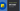# How to convert Map to Array in Javascript / Typescript

09 Aug, 2022, 4 min read

# How to convert Map to Array in Javascript / Typescript

We will look into a quick recipe for converting a javascript map into an array. This can be pretty useful when you are trying to store the values or keys of the map without worrying about the relationship between the key and value

## Convert Map key value to Array in Javascript

A map is a dictionary of key and value pairs. You can use the `map.keys()` method to get a list of keys from the map. Then we can convert it into an array using either the `Array.from` constructor method or use the spread operator

``````let userData = new Map();

userData.set('name', 'Test');
userData.set('age', '24');
userData.set('hobby', 'Blogging')

// Options 1 - Using the Array.from syntax
const userDataKeys = Array.from(userData.keys());
console.log(userDataKeys);

// Options 2 - Using spread operator

Let’s breakdown the steps in the above example for Option 1

1. We are creating a new `Map` variable called `userData`
2. We are assigning a few key-value pair data to the map variable
3. We are getting the list of all the keys that we have set in the map variable using the `[variable].keys()` method
4. We are creating a new array item using the `Array.from()` syntax

Steps for Options 2

1. Follow the same steps 1 to 3 from the previous options
2. We can use the spread operator `...` to convert the return value of the `[variable].keys()` method to array
``````Output:

["name", "age", "hobby"]
["name", "age", "hobby"]``````

## Convert Map values to Array in Javascript

You can use the `map.values()` method to get a list of keys from the map. Then we can convert it into an array using either the `Array.from` constructor method or use the spread operator

``````let userData = new Map();

userData.set('name', 'Test');
userData.set('age', '24');
userData.set('hobby', 'Blogging')

// Options 1 - Using the Array.from syntax
const userDataValues = Array.from(userData.values());
console.log(userDataValues);

// Options 2 - Using spread operator

Let’s breakdown the steps in the above example for Option 1

1. We are creating a new `Map` variable called `userData`
2. We are assigning a few key-value pair data to the map variable
3. We are getting the list of all the keys that we have set in the map variable using the `[variable].values()` method
4. We are creating a new array item using the `Array.from()` syntax

Steps for Options 2

1. Follow the same steps 1 to 3 from the previous options
2. We can use the spread operator `...` to convert the return value of the `[variable].values()` method to array
``````Output:

["Test", "24", "Blogging"]
["Test", "24", "Blogging"]``````

# How to convert Map to Array in Typescript

Let’s look at a quick example of how you can accomplish the same thing using Typescript

``````let userData = new Map<string, string>();

userData.set('name', 'Test');
userData.set('age', '24');
userData.set('hobby', 'Blogging')

// Options 1 - Using the Array.from syntax
const userDataValues: string[] = Array.from(userData.values());
console.log(userDataValues);

// Options 2 - Using spread operator

Since we are storing both the key and value as string data types, we can define the `userData` map variable with `Map<string, string>` to represent that both key and value are string types.

`userData.values()` method will return the value of type `IterableIterator<string>` which can be passed to the `Array.from` or spread operator to convert that into a `string[]` type.

If you want to store the key as a number, you can do the following

``````let userData2 = new Map<number, string>();

userData2.set(1, "Test");

const userDataKeys: number[] = [...userData2.keys()]``````

## Conclusion

Please feel free to reach out to us if you have a better solution or feedback on the post.

If you want to learn more about web technologies, you can check out our Discord server - https://discord.gg/AUjrcK6eep